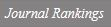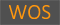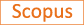Multiplicity of Small or Large Energy Solutions for Kirchhoff-Schrodinger-Type Equations Involving the Fractional p-Laplacian in R-N

Title
Multiplicity of Small or Large Energy Solutions for Kirchhoff-Schrodinger-Type Equations Involving the Fractional p-Laplacian in R-N
Authors
Kim, Jae-MyoungKim, Yun-HoLee, Jongrak
Ewha Authors
Issue Date
2018
Journal Title
SYMMETRY-BASEL
ISSN
2073-8994Citation
SYMMETRY-BASEL vol. 10, no. 10
Keywords
fractional p-LaplacianKirchhoff-type equationsfountain theoremmodified functional methodsMoser iteration method
Publisher
MDPI
Indexed
SCIE; SCOPUSDocument Type
Article
Abstract
We herein discuss the following elliptic equations: M( integral(RN)integral(RN) vertical bar u(x)-u(y)vertical bar(p)/vertical bar x-y vertical bar(N+ps) dx dy)(-Delta)(p)(s)u + V(x)vertical bar u vertical bar(p-2) u = lambda f(x,u) in R-N, where (-Delta)(p)(s) is the fractional p-Laplacian defined by (-Delta)(p)(s) u(x) = 2 lim(epsilon SE arrow 0 )integral(RN\B epsilon(x)) vertical bar u(x)-u(y)vertical bar(p-2)(u(x)-u(y))/vertical bar x-y vertical bar(N+ps) dy, x is an element of R-N. Here, B-epsilon(x): = {y is an element of R-N: vertical bar x-y vertical bar < epsilon}, V : R-N -> (0, infinity) is a continuous function and f : R-N x R -> R is the Caratheodory function. Furthermore, M : R-0(+)-> R+ is a Kirchhoff-type function. This study has two aims. One is to study the existence of infinitely many large energy solutions for the above problem via the variational methods. In addition, a major point is to obtain the multiplicity results of the weak solutions for our problem under various assumptions on the Kirchhoff function M and the nonlinear term f. The other is to prove the existence of small energy solutions for our problem, in that the sequence of solutions converges to 0 in the L-infinity-norm.
DOI
10.3390/sym10100436
Appears in Collections:
연구기관 > 수리과학연구소 > Journal papers
Files in This Item: